## Matrices

#### Introduction

A matrix is a rectangular array of numbers. All of the following are examples of matrices.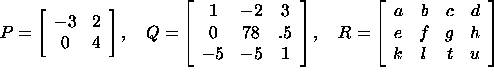Matrices are a useful tool for easily representing large amounts of information and solving multivariable problems. Some situations in which matrices are useful include:

• Representing and solving systems of linear equations
• Solving systems of differential equations
• Simplifying formulas involving 2 or more variables or dimensions (examples include multi-variable statistics formulas and many physical formulas in 2 or more dimensions)

We represent the dimensions of a matrix with m rows and n columns with the notation mxn. The matrices above (from left to right) have dimensions of 2x2, 3x3, and 3x4. One can think of a matrix as being a group of row vectors or column vectors. For the matrix R above, the row vectors are [a b c d], [e f g h], and [k l t u] and the column vectors are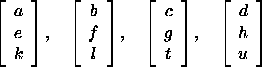The usual two- or three-dimensional vector is typically represented as a single column vector. The elements of a matrix A are frequently labeled as A_ij where i is the number of the row the element is in and j is the number of the column the element is in. For the matrix P above, A_11=-3, A_12=2, A_21=0, and A_22=4.

#### Algebraic Operations Involving Matrices

Matrices can be added, subtracted, and multiplied by scalars in a quite natural manner. For matrices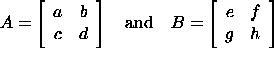we have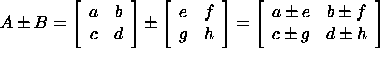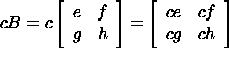The above examples for 2x2 matrices can be generalized quite easily to larger rectangular matrices. Note that two matrices must have the same dimensions in order to be added or subtracted. Matrix addition is clearly commutative and associative (i.e. A+B=B+A and A+(B+C)=(A+B)+C).

#### Example

The sum of the matrices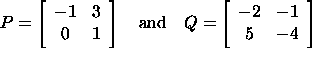is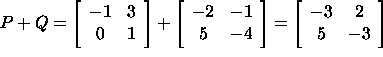It is also possible to multiply two matrices together. The product of two 2x2 matrices is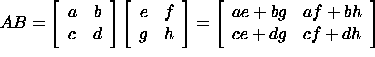The following is an easy way to remember this operation: the element AB_ij of the matrix AB is the dot product of the ith row vector of the matrix A (the matrix on the left) with the jth column vector of the matrix B (the matrix on the right). When generalizing to multiply matrices of different sizes, we must note that to perform the multiplication it is necessary for the matrix on the left to have the same number of columns as the matrix on the right has rows (or else we would not be able to carry out the specified dot product). To summarize, a matrix A with m columns can only be multiplied (on the right) by a matrix B with m rows.

#### Example

Suppose we wish to multiply the matrices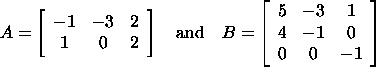The element AB_11 is the dot product of the row vector [-1 -3 2] of A with the column vector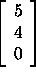of B: AB_11=-1(5)+(-3)(4)+2(0)=-17. If we continue this process and find the other 5 entries of AB we have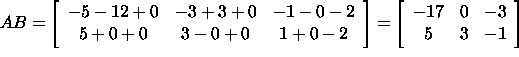ERROR NOTICE:
The middle entry in the top row of AB should be (-1)(-3)+(-3)(-1)+(2)(0)= 6.
The middle entry in the bottom row of AB should be (1)(-3)+(0)(-1)+(2)(0)=-3.

If we reverse the order of the matrices A and B to try to find the product BA we find that the multiplication is not possible. We cannot find the dot product of a row vector of B (with length 3) with a column vector of A (with length 2).

We have discovered another one of the algebraic properties of matrices: non-commutativity of multiplication. As our example above illustrates, the product BA might not be defined even if the product AB is defined. For appropriate choices of A, B, and C (i.e. so the multiplication is defined), the following is a list of commonly used multiplicative matrix properties:

• Non-commutativity: AB is not necessarily equal to BA
• Associativity: A(BC)=(AB)C
• Distributivity: A(B+C)=AB+BC and (A+B)C=AC+BC
• Scalar multiplication: c(AB)=(cA)B=A(cB)